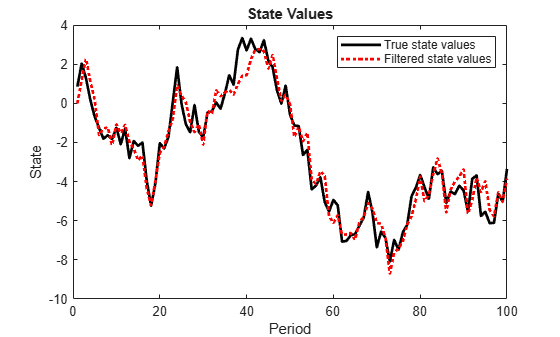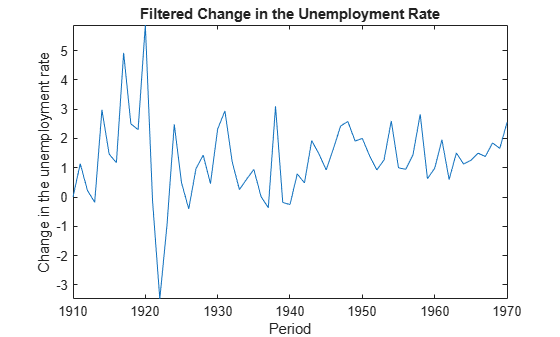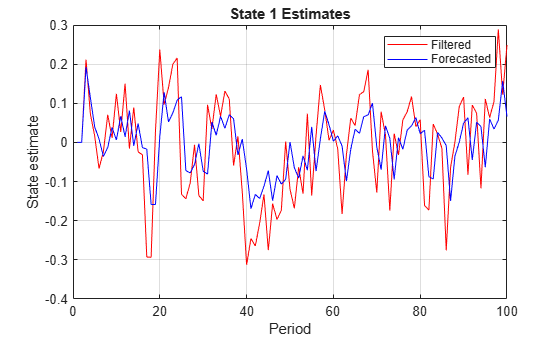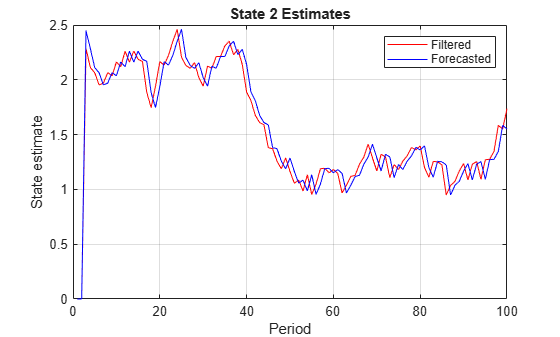# filter

Forward recursion of diffuse state-space models

## Syntax

``X = filter(Mdl,Y)``
``X = filter(Mdl,Y,Name,Value)``
``````[X,logL,Output] = filter(___)``````

## Description

example

````X = filter(Mdl,Y)` returns filtered states (`X`) by performing forward recursion of the fully specified diffuse state-space model `Mdl`. That is, `filter` applies the diffuse Kalman filter using `Mdl` and the observed responses `Y`.```

example

````X = filter(Mdl,Y,Name,Value)` uses additional options specified by one or more `Name,Value` pair arguments. For example, specify the regression coefficients and predictor data to deflate the observations, or specify to use the univariate treatment of a multivariate model.If `Mdl` is not fully specified, then you must specify the unknown parameters as known scalars using the `'``Params``'` `Name,Value` pair argument.```

example

``````[X,logL,Output] = filter(___)``` additionally returns the loglikelihood value (`logL`) and an output structure array (`Output`) using any of the input arguments in the previous syntaxes. `Output` contains: Filtered and forecasted statesEstimated covariance matrices of the filtered and forecasted statesLoglikelihood valueForecasted observations and its estimated covariance matrixAdjusted Kalman gainVector indicating which data the software used to filter ```

## Input Arguments

expand all

Diffuse state-space model, specified as an `dssm` model object returned by `dssm` or `estimate`.

If `Mdl` is not fully specified (that is, `Mdl` contains unknown parameters), then specify values for the unknown parameters using the `'``Params``'` name-value pair argument. Otherwise, the software issues an error. `estimate` returns fully-specified state-space models.

`Mdl` does not store observed responses or predictor data. Supply the data wherever necessary using the appropriate input or name-value pair arguments.

Observed response data, specified as a numeric matrix or a cell vector of numeric vectors.

• If `Mdl` is time invariant with respect to the observation equation, then `Y` is a T-by-n matrix, where each row corresponds to a period and each column corresponds to a particular observation in the model. T is the sample size and m is the number of observations per period. The last row of `Y` contains the latest observations.

• If `Mdl` is time varying with respect to the observation equation, then `Y` is a T-by-1 cell vector. Each element of the cell vector corresponds to a period and contains an nt-dimensional vector of observations for that period. The corresponding dimensions of the coefficient matrices in `Mdl.C{t}` and `Mdl.D{t}` must be consistent with the matrix in `Y{t}` for all periods. The last cell of `Y` contains the latest observations.

`NaN` elements indicate missing observations. For details on how the Kalman filter accommodates missing observations, see Algorithms.

### Name-Value Arguments

Specify optional pairs of arguments as `Name1=Value1,...,NameN=ValueN`, where `Name` is the argument name and `Value` is the corresponding value. Name-value arguments must appear after other arguments, but the order of the pairs does not matter.

Before R2021a, use commas to separate each name and value, and enclose `Name` in quotes.

Example: `'Beta',beta,'Predictors',Z` specifies to deflate the observations by the regression component composed of the predictor data `Z` and the coefficient matrix `beta`.

Regression coefficients corresponding to predictor variables, specified as the comma-separated pair consisting of `'Beta'` and a d-by-n numeric matrix. d is the number of predictor variables (see `Predictors`) and n is the number of observed response series (see `Y`).

If `Mdl` is an estimated state-space model, then specify the estimated regression coefficients stored in `estParams`.

Values for unknown parameters in the state-space model, specified as the comma-separated pair consisting of `'Params'` and a numeric vector.

The elements of `Params` correspond to the unknown parameters in the state-space model matrices `A`, `B`, `C`, and `D`, and, optionally, the initial state mean `Mean0` and covariance matrix `Cov0`.

• If you created `Mdl` explicitly (that is, by specifying the matrices without a parameter-to-matrix mapping function), then the software maps the elements of `Params` to `NaN`s in the state-space model matrices and initial state values. The software searches for `NaN`s column-wise following the order `A`, `B`, `C`, `D`, `Mean0`, and `Cov0`.

• If you created `Mdl` implicitly (that is, by specifying the matrices with a parameter-to-matrix mapping function), then you must set initial parameter values for the state-space model matrices, initial state values, and state types within the parameter-to-matrix mapping function.

If `Mdl` contains unknown parameters, then you must specify their values. Otherwise, the software ignores the value of `Params`.

Data Types: `double`

Predictor variables in the state-space model observation equation, specified as the comma-separated pair consisting of `'Predictors'` and a T-by-d numeric matrix. T is the number of periods and d is the number of predictor variables. Row t corresponds to the observed predictors at period t (Zt). The expanded observation equation is

`${y}_{t}-{Z}_{t}\beta =C{x}_{t}+D{u}_{t}.$`

That is, the software deflates the observations using the regression component. β is the time-invariant vector of regression coefficients that the software estimates with all other parameters.

If there are n observations per period, then the software regresses all predictor series onto each observation.

If you specify `Predictors`, then `Mdl` must be time invariant. Otherwise, the software returns an error.

By default, the software excludes a regression component from the state-space model.

Data Types: `double`

Final period for diffuse state initialization, specified as the comma-separated pair consisting of `'SwitchTime'` and a positive integer. That is, `estimate` uses the observations from period 1 to period `SwitchTime` as a presample to implement the exact initial Kalman filter (see Diffuse Kalman Filter and ). After initializing the diffuse states, `estimate` applies the standard Kalman filter to the observations from periods `SwitchTime` + 1 to T.

The default value for `SwitchTime` is the last period in which the estimated smoothed state precision matrix is singular (i.e., the inverse of the covariance matrix). This specification represents the fewest number of observations required to initialize the diffuse states. Therefore, it is a best practice to use the default value.

If you set `SwitchTime` to a value greater than the default, then the effective sample size decreases. If you set `SwitchTime` to a value that is fewer than the default, then `estimate` might not have enough observations to initialize the diffuse states, which can result in an error or improper values.

In general, estimating, filtering, and smoothing state-space models with at least one diffuse state requires `SwitchTime` to be at least one. The default estimation display contains the effective sample size.

Data Types: `double`

Forecast uncertainty threshold, specified as the comma-separated pair consisting of `'Tolerance'` and a nonnegative scalar.

If the forecast uncertainty for a particular observation is less than `Tolerance` during numerical estimation, then the software removes the uncertainty corresponding to the observation from the forecast covariance matrix before its inversion.

It is best practice to set `Tolerance` to a small number, for example, `le-15`, to overcome numerical obstacles during estimation.

Example: `'Tolerance',le-15`

Data Types: `double`

Univariate treatment of a multivariate series flag, specified as the comma-separated pair consisting of `'Univariate'` and `true` or `false`. Univariate treatment of a multivariate series is also known as sequential filtering.

The univariate treatment can accelerate and improve numerical stability of the Kalman filter. However, all observation innovations must be uncorrelated. That is, DtDt' must be diagonal, where Dt, t = 1,...,T, is one of the following:

• The matrix `D{t}` in a time-varying state-space model

• The matrix `D` in a time-invariant state-space model

Example: `'Univariate',true`

Data Types: `logical`

## Output Arguments

expand all

Filtered states, returned as a numeric matrix or a cell vector of numeric vectors.

If `Mdl` is time invariant, then the number of rows of `X` is the sample size, T, and the number of columns of `X` is the number of states, m. The last row of `X` contains the latest filtered states.

If `Mdl` is time varying, then `X` is a cell vector with length equal to the sample size. Cell t of `X` contains a vector of filtered states with length equal to the number of states in period t. The last cell of `X` contains the latest filtered states.

`filter` pads the first `SwitchTime` periods of `X` with zeros or empty cells. The zeros or empty cells represent the periods required to initialize the diffuse states.

Loglikelihood function value, returned as a scalar.

Missing observations and observations before `SwitchTime` do not contribute to the loglikelihood.

Filtering results by period, returned as a structure array.

`Output` is a T-by-1 structure, where element t corresponds to the filtering result at time t.

• If `Univariate` is `false` (it is by default), then the following table outlines the fields of `Output`.

FieldDescriptionEstimate of
`LogLikelihood`Scalar loglikelihood objective function valueN/A
`FilteredStates`mt-by-1 vector of filtered states$E\left({x}_{t}|{y}_{1},...,{y}_{t}\right)$
`FilteredStatesCov`mt-by-mt variance-covariance matrix of filtered states$Var\left({x}_{t}|{y}_{1},...,{y}_{t}\right)$
`ForecastedStates`mt-by-1 vector of state forecasts$E\left({x}_{t}|{y}_{1},...,{y}_{t-1}\right)$
`ForecastedStatesCov`mt-by-mt variance-covariance matrix of state forecasts$Var\left({x}_{t}|{y}_{1},...,{y}_{t-1}\right)$
`ForecastedObs`ht-by-1 forecasted observation vector$E\left({y}_{t}|{y}_{1},...,{y}_{t-1}\right)$
`ForecastedObsCov`ht-by-ht variance-covariance matrix of forecasted observations$Var\left({y}_{t}|{y}_{1},...,{t}_{t-1}\right)$
`KalmanGain`mt-by-nt adjusted Kalman gain matrixN/A
`DataUsed`ht-by-1 logical vector indicating whether the software filters using a particular observation. For example, if observation i at time t is a `NaN`, then element i in `DataUsed` at time t is `0`.N/A

• If `Univarite` is `true`, then the fields of `Output` are the same as in the previous table, except for the following amendments.

FieldChanges
`ForecastedObs`Same dimensions as if `Univariate = 0`, but only the first elements are equal
`ForecastedObsCov`

n-by-1 vector of forecasted observation variances.

The first element of this vector is equivalent to `ForecastedObsCov(1,1)` when `Univariate` is `false`. The rest of the elements are not necessarily equivalent to their corresponding values in `ForecastObsCov` when `Univariate`.

`KalmanGain`Same dimensions as if `Univariate` is `false`, though `KalmanGain` might have different entries.

`filter` pads the first `SwitchTime` periods of the fields of `Output` with empty cells. These empty cells represent the periods required to initialize the diffuse states.

## Examples

expand all

Suppose that a latent process is a random walk. The state equation is

`${x}_{t}={x}_{t-1}+{u}_{t},$`

where ${u}_{t}$ is Gaussian with mean 0 and standard deviation 1.

Generate a random series of 100 observations from ${x}_{t}$, assuming that the series starts at 1.5.

```T = 100; x0 = 1.5; rng(1); % For reproducibility u = randn(T,1); x = cumsum([x0;u]); x = x(2:end);```

Suppose further that the latent process is subject to additive measurement error. The observation equation is

`${y}_{t}={x}_{t}+{\epsilon }_{t},$`

where ${\epsilon }_{t}$ is Gaussian with mean 0 and standard deviation 0.75. Together, the latent process and observation equations compose a state-space model.

Use the random latent state process (`x`) and the observation equation to generate observations.

`y = x + 0.75*randn(T,1);`

Specify the four coefficient matrices.

```A = 1; B = 1; C = 1; D = 0.75;```

Create the diffuse state-space model using the coefficient matrices. Specify that the initial state distribution is diffuse.

`Mdl = dssm(A,B,C,D,'StateType',2)`
```Mdl = State-space model type: dssm State vector length: 1 Observation vector length: 1 State disturbance vector length: 1 Observation innovation vector length: 1 Sample size supported by model: Unlimited State variables: x1, x2,... State disturbances: u1, u2,... Observation series: y1, y2,... Observation innovations: e1, e2,... State equation: x1(t) = x1(t-1) + u1(t) Observation equation: y1(t) = x1(t) + (0.75)e1(t) Initial state distribution: Initial state means x1 0 Initial state covariance matrix x1 x1 Inf State types x1 Diffuse ```

`Mdl` is an `dssm` model. Verify that the model is correctly specified using the display in the Command Window.

Filter states for periods 1 through 100. Plot the true state values and the filtered state estimates.

```filteredX = filter(Mdl,y); figure plot(1:T,x,'-k',1:T,filteredX,':r','LineWidth',2) title({'State Values'}) xlabel('Period') ylabel('State') legend({'True state values','Filtered state values'})```The true values and filter estimates are approximately the same, except for the first filtered state, which is zero.

Suppose that the linear relationship between unemployment rate and the nominal gross national product (nGNP) is of interest. Suppose further that unemployment rate is an AR(1) series. Symbolically, and in state-space form, the model is

`$\begin{array}{l}{x}_{t}=\varphi {x}_{t-1}+\sigma {u}_{t}\\ {y}_{t}-\beta {Z}_{t}={x}_{t},\end{array}$`

where:

• ${x}_{t}$ is the unemployment rate at time t.

• ${y}_{t}$ is the observed change in the unemployment rate being deflated by the return of nGNP (${Z}_{t}$).

• ${u}_{t}$ is the Gaussian series of state disturbances having mean 0 and unknown standard deviation $\sigma$.

Load the Nelson-Plosser data set, which contains the unemployment rate and nGNP series, among other things.

`load Data_NelsonPlosser`

Preprocess the data by taking the natural logarithm of the nGNP series, and removing the starting `NaN` values from each series.

```isNaN = any(ismissing(DataTable),2); % Flag periods containing NaNs gnpn = DataTable.GNPN(~isNaN); y = diff(DataTable.UR(~isNaN)); T = size(gnpn,1); % The sample size Z = price2ret(gnpn);```

This example continues using the series without `NaN` values. However, using the Kalman filter framework, the software can accommodate series containing missing values.

Specify the coefficient matrices.

```A = NaN; B = NaN; C = 1;```

Create the state-space model using `dssm` by supplying the coefficient matrices and specifying that the state values come from a diffuse distribution. The diffuse specification indicates complete ignorance about the moments of the initial distribution.

```StateType = 2; Mdl = dssm(A,B,C,'StateType',StateType);```

Estimate the parameters. Specify the regression component and its initial value for optimization using the `'Predictors'` and `'Beta0'` name-value pair arguments, respectively. Display the estimates and all optimization diagnostic information. Restrict the estimate of $\sigma$ to all positive, real numbers.

```params0 = [0.3 0.2]; % Initial values chosen arbitrarily Beta0 = 0.1; [EstMdl,estParams] = estimate(Mdl,y,params0,'Predictors',Z,'Beta0',Beta0,... 'lb',[-Inf 0 -Inf]);```
```Method: Maximum likelihood (fmincon) Effective Sample size: 60 Logarithmic likelihood: -110.477 Akaike info criterion: 226.954 Bayesian info criterion: 233.287 | Coeff Std Err t Stat Prob -------------------------------------------------------- c(1) | 0.59436 0.09408 6.31738 0 c(2) | 1.52554 0.10758 14.17991 0 y <- z(1) | -24.26161 1.55730 -15.57930 0 | | Final State Std Dev t Stat Prob x(1) | 2.54764 0 Inf 0 ```

`EstMdl` is an `ssm` model, and you can access its properties using dot notation.

Filter the estimated diffuse state-space model. `EstMdl` does not store the data or the regression coefficients, so you must pass in them in using the name-value pair arguments `'Predictors'` and `'Beta'`, respectively. Plot the estimated, filtered states.

```filteredX = filter(EstMdl,y,'Predictors',Z,'Beta',estParams(end)); figure plot(dates(end-(T-1)+1:end),filteredX); xlabel('Period') ylabel('Change in the unemployment rate') title('Filtered Change in the Unemployment Rate') axis tight```Estimate a diffuse state-space model, filter the states, and then extract other estimates from the `Output` output argument.

Consider the diffuse state-space model

`$\begin{array}{l}\left[\begin{array}{c}{x}_{1,t}\\ {x}_{2,t}\end{array}\right]=\left[\begin{array}{cc}\varphi & 0\\ 0& 1\end{array}\right]\left[\begin{array}{c}{x}_{1,t-1}\\ {x}_{2,t-1}\end{array}\right]+\left[\begin{array}{cc}{\sigma }_{1}& 0\\ 0& {\sigma }_{2}\end{array}\right]\left[\begin{array}{c}{u}_{1,t}\\ {u}_{2,t}\end{array}\right]\\ {y}_{t}=\left[\begin{array}{cc}1& 1\end{array}\right]\left[\begin{array}{c}{x}_{1,t}\\ {x}_{2,t}\end{array}\right]\end{array}$`

The state variable ${x}_{1,t}$ is an AR(1) model with autoregressive coefficient $\varphi$. ${x}_{2,t}$ is a random walk. The disturbances ${u}_{1,t}$ and ${u}_{2,t}$ are independent Gaussian random variables with mean 0 and standard deviations ${\sigma }_{1}$ and ${\sigma }_{2}$, respectively. The observation ${y}_{t}$ is the error-free sum of ${x}_{1,t}$ and ${x}_{2,t}$.

Generate data from the state-space model. To simulate the data, suppose that the sample size $T=100$, $\varphi =0.6$, ${\sigma }_{1}=0.2$, ${\sigma }_{2}=0.1$, and ${x}_{1,0}={x}_{2,0}=2$.

```rng(1); % For reproducibility T = 100; ARMdl = arima('AR',0.6,'Constant',0,'Variance',0.2^2); x1 = simulate(ARMdl,T,'Y0',2); u3 = 0.1*randn(T,1); x3 = cumsum([2;u3]); x3 = x3(2:end); y = x1 + x3;```

Specify the coefficient matrices of the state-space model. To indicate unknown parameters, use `NaN` values.

```A = [NaN 0; 0 1]; B = [NaN 0; 0 NaN]; C = [1 1];```

Create a diffuse state-space model that describes the model above. Specify that ${x}_{1,t}$ and ${x}_{2,t}$ have diffuse initial state distributions.

```StateType = [2 2]; Mdl = dssm(A,B,C,'StateType',StateType);```

Estimate the unknown parameters of `Mdl`. Choose initial parameter values for optimization. Specify that the standard deviations are constrained to be positive, but all other parameters are unconstrained using the `'lb'` name-value pair argument.

```params0 = [0.01 0.1 0.01]; % Initial values chosen arbitrarily EstMdl = estimate(Mdl,y,params0,'lb',[-Inf 0 0]);```
```Method: Maximum likelihood (fmincon) Effective Sample size: 98 Logarithmic likelihood: 3.44283 Akaike info criterion: -0.885655 Bayesian info criterion: 6.92986 | Coeff Std Err t Stat Prob -------------------------------------------------- c(1) | 0.54134 0.20494 2.64145 0.00826 c(2) | 0.18439 0.03305 5.57897 0 c(3) | 0.11783 0.04347 2.71039 0.00672 | | Final State Std Dev t Stat Prob x(1) | 0.24884 0.17168 1.44943 0.14722 x(2) | 1.73762 0.17168 10.12121 0 ```

The parameters are close to their true values.

Filter the states of `EstMdl`, and request all other available output.

`[X,logL,Output] = filter(EstMdl,y);`

`X` is a `T`-by-2 matrix of filtered states, `logL` is the final optimized log-likelihood value, and `Output` is a structure array containing various estimates that the Kalman filter requires. List the fields of `output` using `fields`.

`fields(Output)`
```ans = 9x1 cell {'LogLikelihood' } {'FilteredStates' } {'FilteredStatesCov' } {'ForecastedStates' } {'ForecastedStatesCov'} {'ForecastedObs' } {'ForecastedObsCov' } {'KalmanGain' } {'DataUsed' } ```

Convert `Output` to a table.

```OutputTbl = struct2table(Output); OutputTbl(1:10,1:5) % Display first ten rows of first five variables```
```ans=10×5 table LogLikelihood FilteredStates FilteredStatesCov ForecastedStates ForecastedStatesCov _____________ ______________ _________________ ________________ ___________________ {0x0 double} {0x0 double} {0x0 double} {0x0 double} {0x0 double} {0x0 double} {0x0 double} {0x0 double} {0x0 double} {0x0 double} {[ 0.1827]} {2x1 double} {2x2 double} {2x1 double} {2x2 double} {[ 0.0972]} {2x1 double} {2x2 double} {2x1 double} {2x2 double} {[ 0.4472]} {2x1 double} {2x2 double} {2x1 double} {2x2 double} {[ 0.2073]} {2x1 double} {2x2 double} {2x1 double} {2x2 double} {[ 0.5167]} {2x1 double} {2x2 double} {2x1 double} {2x2 double} {[ 0.2389]} {2x1 double} {2x2 double} {2x1 double} {2x2 double} {[ 0.5064]} {2x1 double} {2x2 double} {2x1 double} {2x2 double} {[ -0.0105]} {2x1 double} {2x2 double} {2x1 double} {2x2 double} ```

The first two rows of the table contain empty cells or zeros. These correspond to the observations required to initialize the diffuse Kalman filter. That is, `SwitchTime` is 2.

`SwitchTime = 2;`

Plot the filtered and forecasted states.

```ForeX = cell2mat(OutputTbl.ForecastedStates')'; % Orient forecasted states ForeX = [zeros(SwitchTime,2);ForeX]; % Include zeros for initialization figure; plot(1:T,X(:,1),'r',1:T,ForeX(:,1),'b'); xlabel('Period'); ylabel('State estimate'); title('State 1 Estimates') legend('Filtered','Forecasted'); grid on;``````figure; plot(1:T,X(:,2),'r',1:T,ForeX(:,2),'b'); xlabel('Period'); ylabel('State estimate'); title('State 2 Estimates') legend('Filtered','Forecasted'); grid on;```## Tips

• `Mdl` does not store the response data, predictor data, and the regression coefficients. Supply the data wherever necessary using the appropriate input or name-value pair arguments.

• It is a best practice to allow `filter` to determine the value of `SwitchTime`. However, in rare cases, you might experience numerical issues during estimation, filtering, or smoothing diffuse state-space models. For such cases, try experimenting with various `SwitchTime` specifications, or consider a different model structure (e.g., simplify or reverify the model). For example, convert the diffuse state-space model to a standard state-space model using `ssm`.

• To accelerate estimation for low-dimensional, time-invariant models, set `'Univariate',true`. Using this specification, the software sequentially updates rather then updating all at once during the filtering process.

## Algorithms

• The Kalman filter accommodates missing data by not updating filtered state estimates corresponding to missing observations. In other words, suppose there is a missing observation at period t. Then, the state forecast for period t based on the previous t – 1 observations and filtered state for period t are equivalent.

• For explicitly defined state-space models, `filter` applies all predictors to each response series. However, each response series has its own set of regression coefficients.

• The diffuse Kalman filter requires presample data. If missing observations begin the time series, then the diffuse Kalman filter must gather enough nonmissing observations to initialize the diffuse states.

• For diffuse state-space models, `filter` usually switches from the diffuse Kalman filter to the standard Kalman filter when the number of cumulative observations and the number of diffuse states are equal. However, if a diffuse state-space model has identifiability issues (e.g., the model is too complex to fit to the data), then `filter` might require more observations to initialize the diffuse states. In extreme cases, `filter` requires the entire sample.

 Durbin J., and S. J. Koopman. Time Series Analysis by State Space Methods. 2nd ed. Oxford: Oxford University Press, 2012.